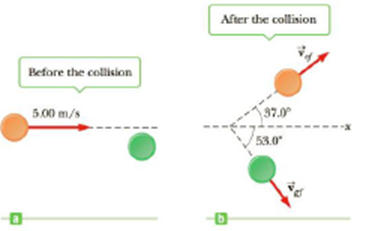Chapter 6, Problem 40P

Chapter
Section
Textbook Problem

Two shuffleboard disks of equal mass, one orange and the other green, are involved in a perfectly elastic glancing collision. The green disk is initially at rest and is struck by the orange disk moving initially to the right at 5.00 m/s as in Figure P6.40a. After the collision, the orange disk moves in a direction that make an angle of 37.0° with the horizontal axis while the green disk makes an angle of 53.0° with this axis as in Figure P6.40b. Determine the speed of each disk after the collision.Figure P6.40

To determine
The speed of the orange disk and green disk after collision.

Explanation

Explanation

Given Info:

The mass of both disc are same, the initial speed of the orange disk is 5.00ms1 , initial speed of the green disk is zero, the direction of orange disk with respect to horizontal is

According to conservation of momentum, the momentum before collision of the disks is equal to momentum after collision.

Consider the components of velocity along positive axis taken as positive and negative axis will taken as negative.

Figure below shows velocity components of orange and green disks after collision.

Apply conservation of momentum along x-axis

mvo=mvofcos37°+mvgfsin53.0°vo=vofcos37°+vgfcos53.0° (I)

• m is the mass of both green and orange disks
• vo is the initial speed of the orange disk
• vof is the final speed of the orange disk
• vgf is the final speed of the green disk

Apply conservation of momentum along y-axis.

0=mvofsin37.0°mvgfsin53.0°vofsin37.0°=vgfsin53.0°vof=vgf(sin53.0°sin37.0°) (II)

Substitute (II) in (I) and 5.00ms1 for vo to calculate vof .

5

Still sussing out bartleby?

Check out a sample textbook solution.

See a sample solution

The Solution to Your Study Problems

Bartleby provides explanations to thousands of textbook problems written by our experts, many with advanced degrees!

Get Started

Give each of the following amines an IUPAC name: a. b. c.

Chemistry for Today: General, Organic, and Biochemistry

What are the components involved?

Chemistry for Engineering Students

Why do giant stars have such low overall density?

Foundations of Astronomy (MindTap Course List)

What biological evidence supports plate tectonics theory?

Oceanography: An Invitation To Marine Science, Loose-leaf Versin